top of page

## Hybrid ICP

Kamil Dreczkowski and Edward Johns

Published at IROS 2021

[Paper]          [Supplementary Material]          [[Code]

Abstract

ICP algorithms typically involve a fixed choice of data association method and a fixed choice of error metric. In this paper, we propose Hybrid ICP, a novel and flexible ICP variant which dynamically optimises both the data association method and error metric based on the live image of an object and the current ICP estimate. We show that when used for object pose estimation, Hybrid ICP is more accurate and more robust to noise than other commonly used ICP variants. We also consider the setting where ICP is applied sequentially with a moving camera, and we study the trade-off between the accuracy of each ICP estimate and the number of ICP estimates available within a fixed amount of time.

Video

## 8-Minute Summary

Quick summary

Existing ICP algorithms use a fixed data association method and fixed error metric. For this reason, they commonly fail to estimate object poses robustly. This is because they are not flexible enough to cope with a range of geometries and ICP initialisations. In this project, we propose Hybrid ICP, a flexible algorithm that uses a live depth image of an object and the current ICP estimate to optimise the choice of the data association method used by ICP. It then conditions the choice of the error metric on the chosen data association method.

In the three GIFs below, the left column illustrates ICP initialisation. The remaining three columns compare Hybrid ICP to some of the most used ICP variants. The error metric used is the Visible Surface Discrapancy (VSD).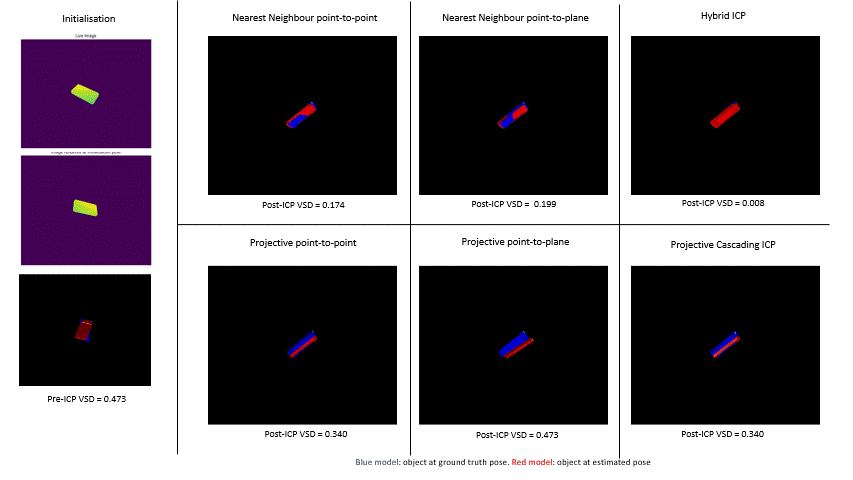Pose estimation refinement with ICP

​Pose estimation is crucial for robots to interact with their surroundings efficiently as it enables them to plan and reason about 3D space. Recently, the 2020 BOP challenge revealed the importance of ICP for accurate pose estimation. This was done by showing that 7 out of 10 top-performing methods relied on ICP for pose estimation refinement. The GIF below shows the results from this challenge, with the top-performing methods that use ICP highlighted in yellow. It also shows the improvement in the average performance of four different methods that resulted from introducing ICP.## ​

ICP is an iterative algorithm for registering two point clouds given an initial estimate of their relative pose. ICP algorithms have two main design choices that largely influence their performance. The first is the choice of the data association method, and the second is the choice of the error metric.

Consider the object model point cloud O and the object visible surface point cloud C, where C is computed from a live depth image of the object. Each iteration of ICP commonly begins by transforming O into the frame of reference of C using the current ICP estimate.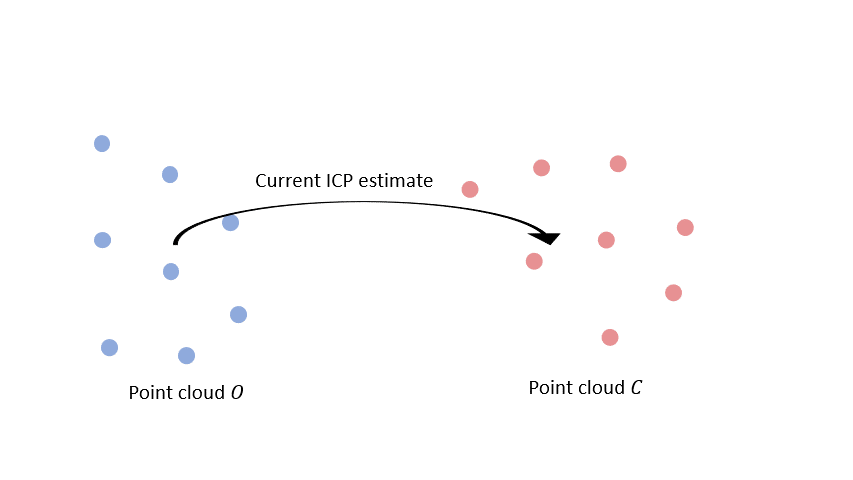## ​Then, a data association method is used to find correspondences between the two point clouds.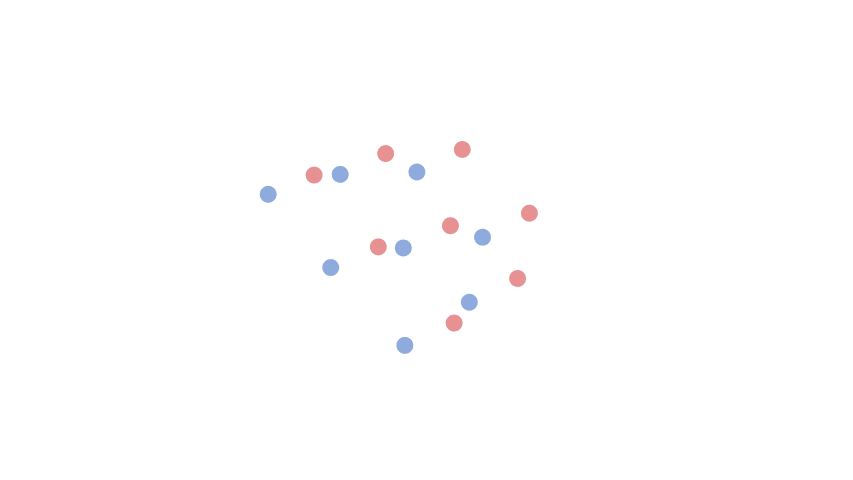Finally, all correspondences are used to minimise some error metric. The output is an incremental transformation that is used to update the current ICP estimate before it moves to the next iteration.

Dynamic Switching - Optimising the choice of the data association method

The two most commonly used data association methods are Nearest Neighbour (NN) and projective data associations.

Nearest Neighbour Data Association

After transforming all model points from the frame of reference of O to that of C, NN data association associates each point with its closest neighbour. The GIF below shows this for a single model point.The pros and cons of algorithms that use NN data association are shown below.Projective Data Association

Algorithms that use projective data association store point clouds O and C in vertex maps. At each pixel location, a vertex map stores the coordinates of the 3D point that was projected to that same pixel in the depth image used to compute it. Note that the object model vertex map is obtained by rendering the object at ICP initialisation.A single 3D point from the object model vertex map is associated with another point by projecting it onto the image plane of the object visible surface vertex map using the camera intrinsic matrix. The 3D point stored at the pixel location to which it is projected onto is then associated with it.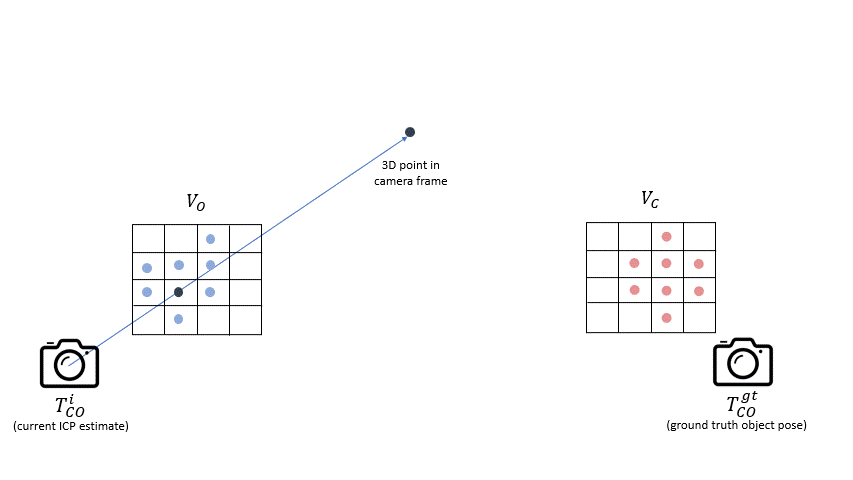The pros and cons of algorithms the use projective data association are shown below.Dynamic Switching

We propose Dynamic Switching to overcome the limitations of NN and projective data association. This simple method uses an estimate of the Visible Surface Discrapancy (VSD) for the current ICP estimate, which we refer to as the MVE, to optimise the choice of the data association method. If the MVE is smaller than some threshold, projective data association is chosen. This is because we know that the object rendered at the current ICP estimate is well aligned with the live depth image and that projective data association will yield many matches. And if the MVE is larger than that threshold, NN data association is chosen. A diagram of Dynamic Switching is shown below.Cascading ICP - Optimising the choice of the error metric

The two most commonly used error metrics are the point-to-point and the point-to-plane error metric. The point-to-point error metric computes the sum of squared distances between all correspondences. In contrast, the point-to-plane error metric computes the sum of squared distances between all points in one point cloud and linear surface approximations in the other. The pros and cons of both error metrics are shown below.To overcome the individual limitations of the two error metrics, we propose Cascading ICP, that first minimises the point-to-point and then the point-to-plane error metric. By first minimising the point-to-point error metric, it brings the two input point clouds into better alignment and decreases the probability of point-to-plane ICP diverging. Then, it refines the result with point-to-plane ICP. A schematic diagram of cascading ICP is shown below.Hybrid ICP - Combining Dynamic Switching, Cascading ICP and Point-to-Point ICP

We combine Dynamic Switching, Cascading ICP and the point-to-point error metric into a single algorithm that we call Hybrid ICP. Hybrid ICP is an iterative algorithm that encapsulates the generic ICP algorithm. During each iteration, dynamic switching is used to optimise the choice of the data association method. If projective data association is chosen, Hybrid ICP aligns the two input point clouds with Cascading ICP for N iterations. If nearest neighbour data association is chosen, it aligns the two input point clouds with point-to-point ICP for N iterations. In this work, we fix the number of hybrid ICP iterations to two. The image below illustrates a single iteration of Hybrid ICP.Results

We consider 20 different objects that are shown in the image below. The ten objects in the top row of the figure were used to tune the hyperparameter of Dynamic Switching, while the ten objects in the bottom row were used for evaluation.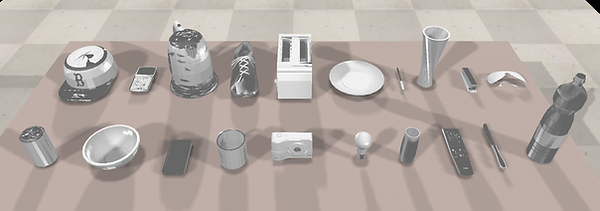We conduct three different experiments to evaluate Hybrid ICP. The first benchmarks its robustness to initialisation noise, the second to noise in depth images, and the third to noise in object models. The performance of Hybrid ICP and two top-performing baselines are shown in the figures below. As these graphs illustrate, Hybrid ICP is more robust to noise than the top-performing baselines.

Robustness to initalisation noiseRobustness to depth image noiseRobustness to model noiseSequential ICP

We also study the setting in which a camera is moving into an object while sequentially re-estimating its pose. An example of a camera moving into a light bulb is shown below.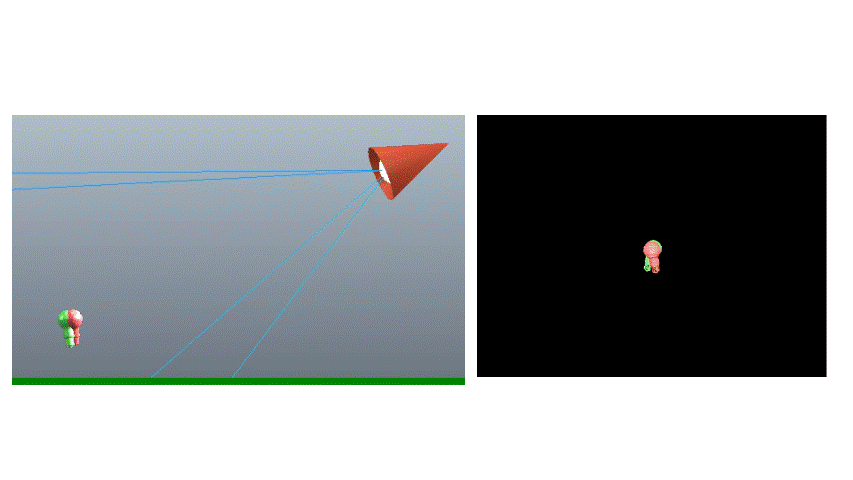In this setting, we not only investigate various methods for fusing SE(3) estimates along a trajectory to get ICP initialisation and the final estimate, but we also investigate the trade-off between each estimate's accuracy and computation time. We do this by considering two underlying ICP algorithms, i.e. an algorithm that is slower but more accurate (Hybrid ICP), and an algorithm that is faster but less accurate (projective cascading ICP). We conclude that none of the considered methods for fusing sequential ICP estimates consistently outperforms the remaining methods and that the choice of the underlying ICP algorithm is strongly dependant on the accuracy of the global pose estimator used for ICP initialisation.